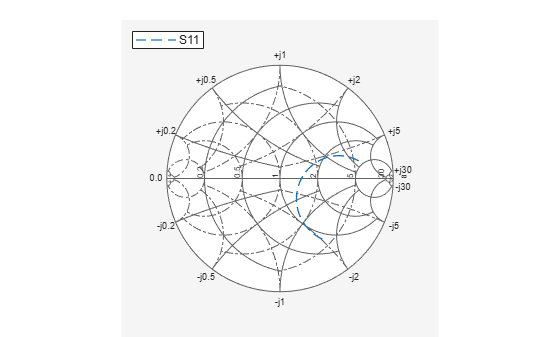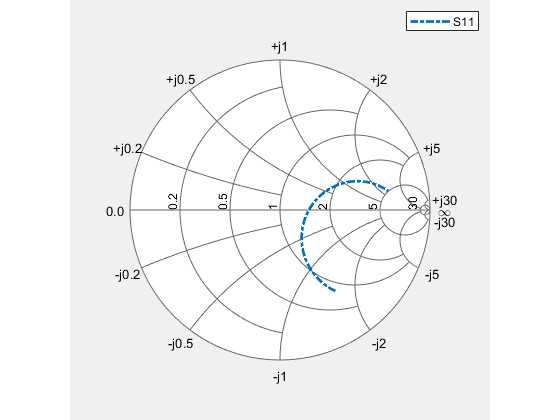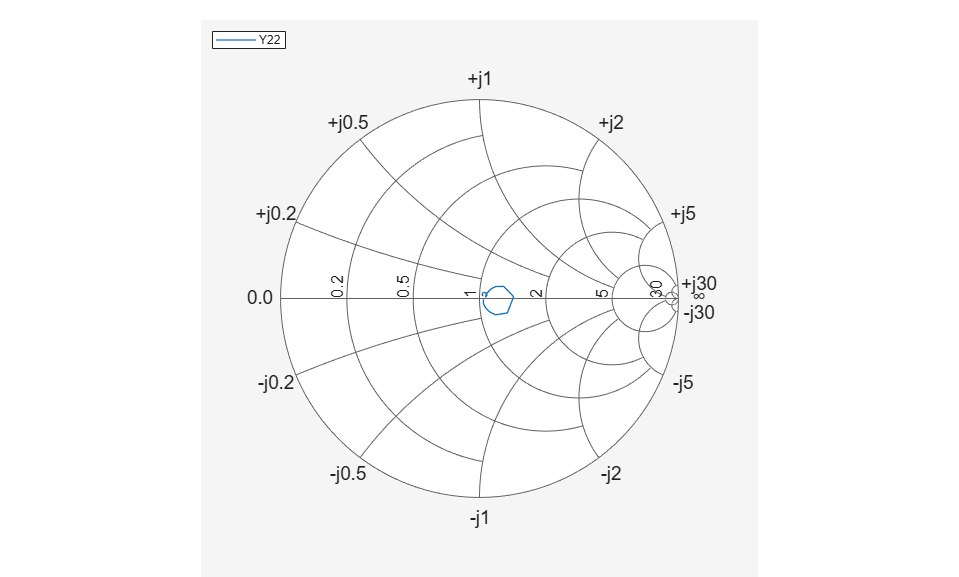# smithplot

Plot measurement data on Smith chart

## Syntax

``smithplot(data)``
``smithplot(frequency,data)``
``smithplot(ax,___)``
``smithplot(hnet)``
``smithplot(hnet,i,j)``
``smithplot(hnet,[i1,j1;i2,j2;....,in,jn])``
``s = smithplot(___)``
``s = smithplot('gco')``
``smithplot(___,Name,Value)``

## Description

example

````smithplot(data)` creates a Smith chart based on input data values. NoteThe Smith chart is commonly used to display the relationship between a reflection coefficient, typically given as S11 or S22, and a normalized impedance. ```
````smithplot(frequency,data)` creates a Smith chart based on frequency and data values.```
````smithplot(ax,___)` creates a Smith chart with a user defined axes handle, `ax`, instead of the current axes handle. Axes handles are not supported for network parameter objects. This parameter can be used with either of the two previous syntaxes.```

````smithplot(hnet)` plots all the network parameter objects in `hnet`.```

````smithplot(hnet,i,j)` plots the (i, j)th parameter of `hnet`. `hnet` is a network parameter object.```

````smithplot(hnet,[i1,j1;i2,j2;....,in,jn])` plots multiple parameters (i1, j1, i2, j2, …, in, jn) of `hnet`. `hnet` is a network parameter object.```
````s = smithplot(___)` returns a Smith chart object handle so you can customize the plot and add measurements. ```
````s = smithplot('gco')` returns a Smith chart object handle of the current plot. This syntax is useful when the function handle, `p` was not returned or retained.```
````smithplot(___,Name,Value)` creates a Smith chart with additional properties specified by one or more name-value pair arguments. `Name` is the property name and `Value` is the corresponding property value. You can specify several name-value pair arguments in any order as `Name1`, `Value1`, `...`, `NameN`, `ValueN`. Properties not specified retain their default values.For list of properties, see SmithPlot Properties (RF Toolbox). NoteThe property `'Parent'` might be used to control the location where Smith chart gets plotted. Target can be figure, UI figure, UI panel, etc. ```

## Examples

collapse all

Create a strip dipole antenna on the Y-Z plane. Calculate the complex S-parameters of the dipole antenna from 60 MHz to 90 MHz, with an interval of 150 kHz.

```d = dipole; freq = linspace(60e6,90e6,200); s = sparameters(d,freq);```

Plot the S11 on a Smith chart.

```hg = smithplot(s,1,1,'GridType','ZY'); hg.LineStyle = '--'``````hg = smithplot with properties: Data: [200x1 double] Frequency: [200x1 double] Show all properties, methods ```

Use the Smith chart interactive menu for changing line and marker styles.

Plot the Smith chart of S-parameters of dipole `d`.

`smithplot(s)`

Right click on the S11 line to reveal interactive menu, `DATASET 1. `Set Line style to '-.' and Properties...>Line Width to '2' to change the line style and width of S11 line on the Smith chart.You can see the changes you made on the Smith chart.```amp = read(rfckt.amplifier,'default.s2p'); Sa = sparameters(amp); ```

Convert the S-parameter data to Y-parameter and plot it on a Smith plot.

```Ya = yparameters(Sa); smithplot(Ya,2,2) ```Add Z-parameter data to the same plot.

```Za = zparameters(Sa); Z12 = rfparam(Za,1,2); Freq = Za.Frequencies; s = smithplot('gco'); add(s, Freq, Z12); ```## Input Arguments

collapse all

Input data, specified as a complex vector or complex matrix.

For a matrix D, the columns of D are independent data sets. For N-by-D arrays, dimensions 2 and greater are independent data sets.

Data Types: `double`
Complex Number Support: Yes

Frequency data, specified as a real vector.

Data Types: `double`

Input objects, specified as a network parameter object.

Data Types: `double`

RF budget object, specified as a `rfbudget` (RF Toolbox) object.

## Output Arguments

collapse all

Smith chart object handle. You can use the handle to customize the plot and add measurements using MATLAB® commands.

## Tips

• To list all the property `Name,Value` pairs in `smithplot`, use `details(s)`. You can use the properties to extract any data from the Smith chart. For example, `s = smithplot(data,'GridType','Z')` displays the impedance data grid from the Smith chart.

• For a list of properties of `smithplot`, see SmithPlot Properties (RF Toolbox).

• You can use the `smithplot` interactive menu to change the line and marker styles.

## Version History

Introduced in R2017b